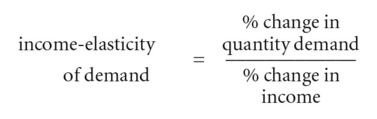# income-elasticity of demand

## income-elasticity of demand

see ELASTICITY OF DEMAND.

## income-elasticity of demand

a measure of the degree of responsiveness of DEMAND to a given change in INCOME:If a given change in income results in a more than proportional change in quantity demanded, then demand is said to be income-elastic, while if a given change in income results in a less than proportional change in quantity demanded, then demand is income-inelastic.

Income-elasticity is positive for a NORMAL PRODUCT and negative for an INFERIOR PRODUCT. STAPLE PRODUCTS tend to have an income-elasticity of demand of less than 1, whereas LUXURY PRODUCTS generally tend to have an income-elasticity of more than 1. See ENGEL'S LAW, INCOME EFFECT, INCOME-CONSUMPTION CURVE, DEMAND CURVE ( SHIFT IN).

Site: Follow: Share:
Open / Close